# Lines and AnglesPage 1

#### WATCH ALL SLIDES

Slide 1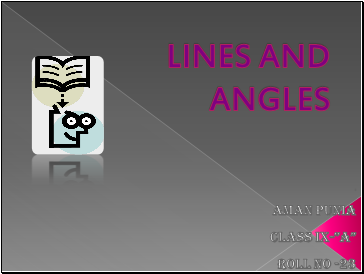LINES AND ANGLES

:

AMAN PUNIA

CLASS IX-”A”

ROLL NO -23

Slide 2## Parallel lines

Def: line that do not intersect.

Illustration:

Notation: l || m AB || CD

Slide 3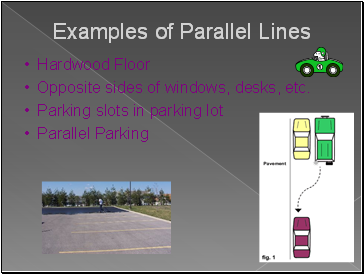## Examples of Parallel Lines

Hardwood Floor

Opposite sides of windows, desks, etc.

Parking slots in parking lot

Parallel Parking

Slide 4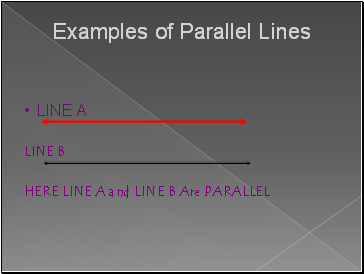Examples of Parallel Lines

LINE A

LINE B

HERE LINE A and LINE B Are PARALLEL

Slide 5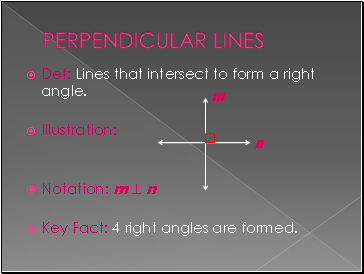## Perpendicular lines

Def: Lines that intersect to form a right angle.

Illustration:

Notation: m  n

Key Fact: 4 right angles are formed.

Slide 6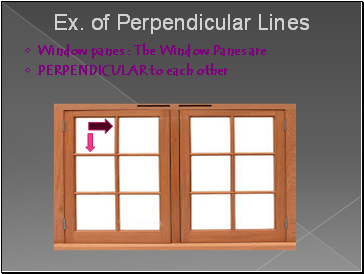Ex. of Perpendicular Lines

Window panes : The Window Panes are

PERPENDICULAR to each other

Slide 7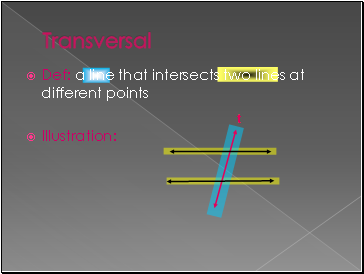## Transversal

Def: a line that intersects two lines at different points

Illustration:

t

Slide 8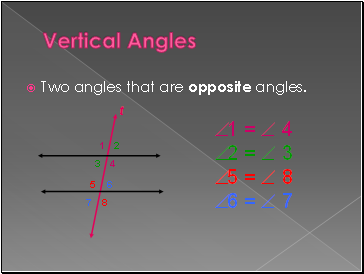## Vertical Angles

Two angles that are opposite angles.

1

2

3

4

5

6

7

8

t

1 =  4

2 =  3

5 =  8

6 =  7

Slide 9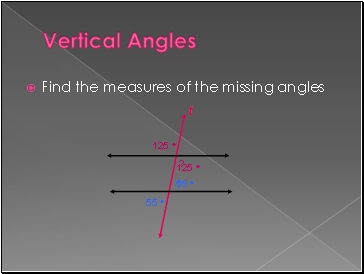Vertical Angles

Find the measures of the missing angles

125 

?

?

55 

t

55 

125 

Slide 10## Supplementary Angles/ Linear Pair

Two angles that form a line (sum=180)

5+6=180

6+8=180

8+7=180

7+5=180

1+2=180

2+4=180

4+3=180

3+1=180

Slide 11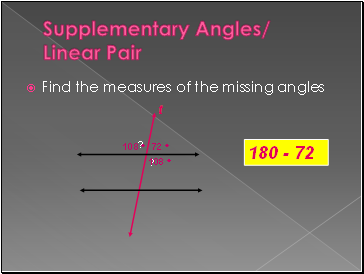Supplementary Angles/ Linear Pair

Find the measures of the missing angles

?

72 

?

t

108 

108 

180 - 72

Slide 12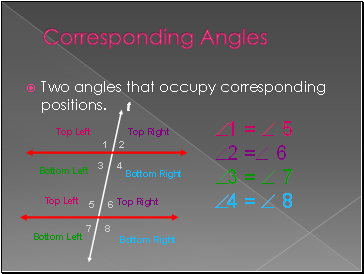## Corresponding Angles

Two angles that occupy corresponding positions.

Top Left

t

Top Left

Top Right

Top Right

Bottom Right

Bottom Right

Bottom Left

Bottom Left

1 =  5

Go to page:
1  2